# Subtract up to 4-digit numbers

## Learning focus

Learn how to subtract up to two four-digit numbers.

This lesson includes:

• a catch-up quiz

• one video

• three slideshows

# Quiz

To get started, let's see how well you know this topic already. Take the catch-up quiz below to find out.

# Learn

Numbers can be subtracted using the column method.

Watch this video to learn about subtracting 4-digit numbers using the column method.

Numbers can be subtracted using the column method.

Let's have a look at using this method to subtract one 3-digit number from another.

## Example 1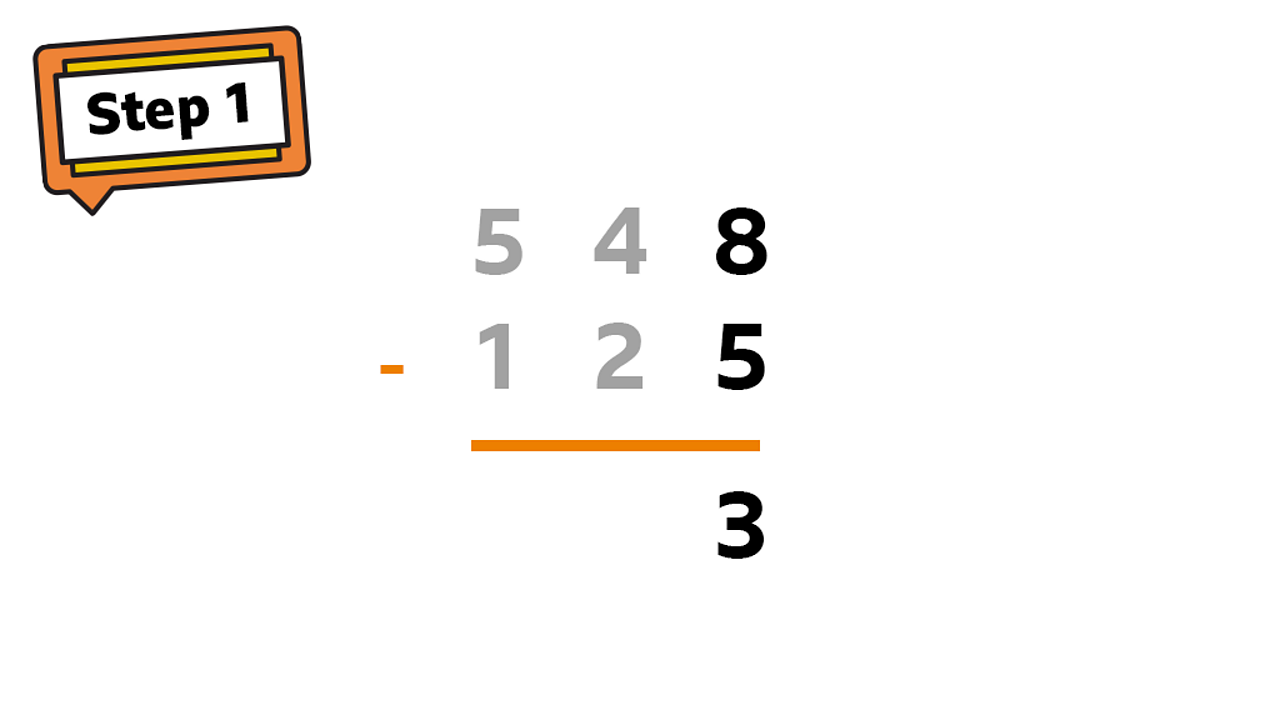Start from the ones column: 8 - 5 = 31 of 4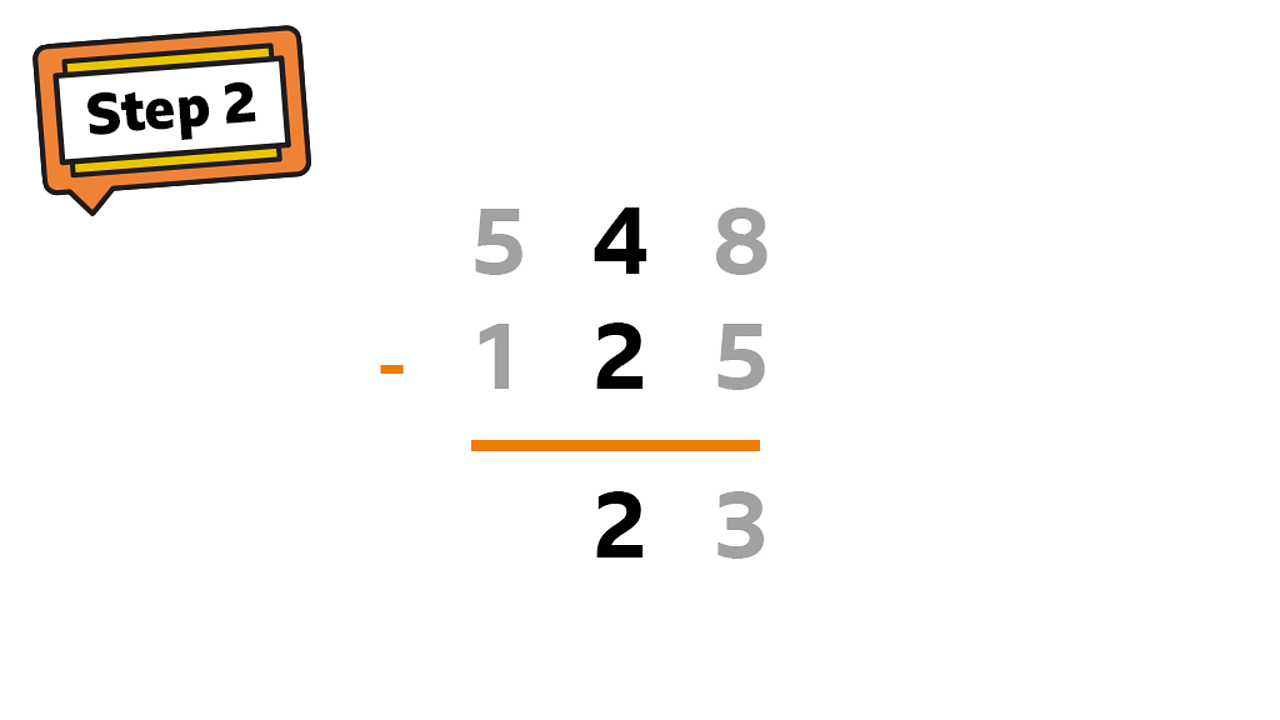In the tens column: 40 - 20 = 20. 2 Is written in tens column as this is worth 202 of 4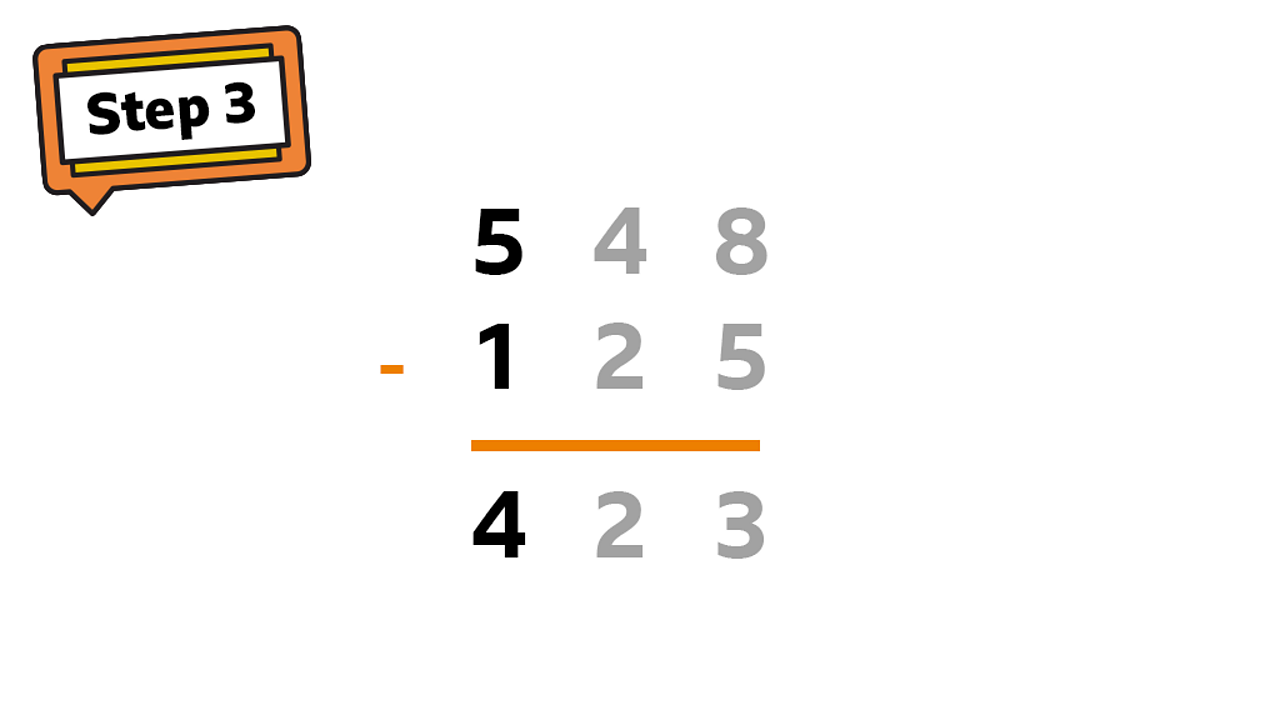In the hundreds column: 500 - 100 = 400. 4 is written in the hundreds column as this is worth 4003 of 4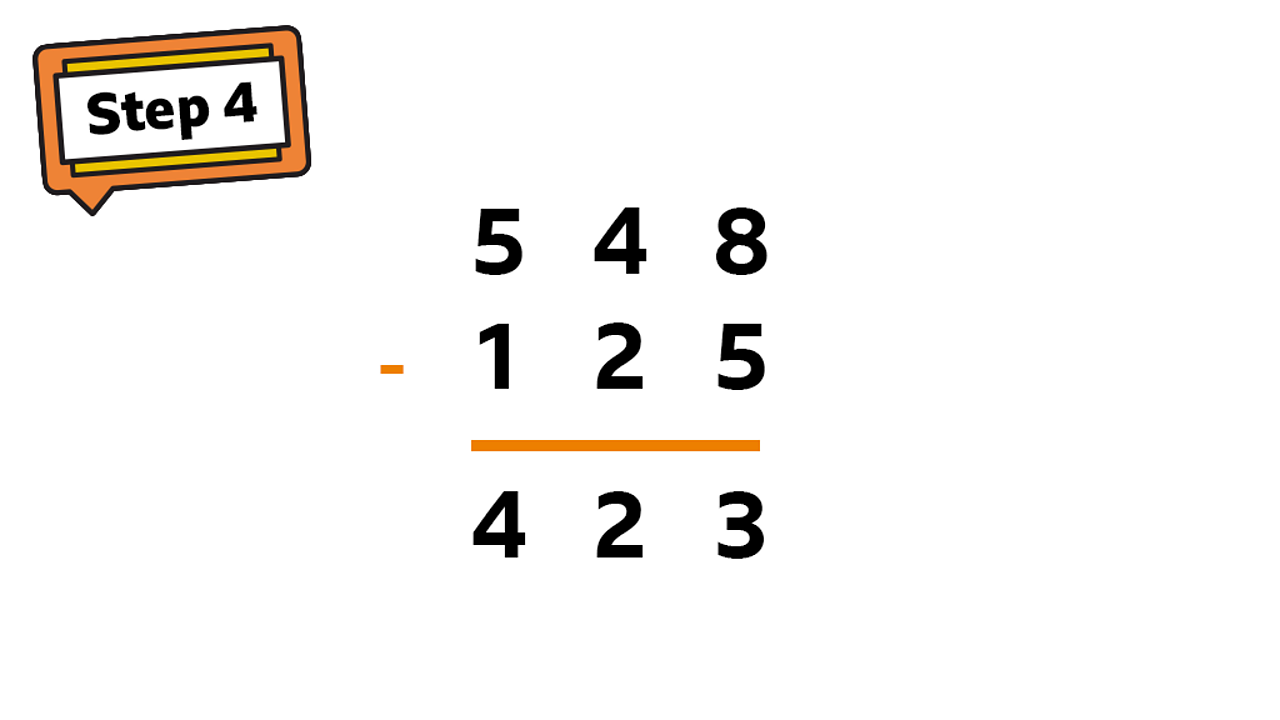The finished subtraction - 4234 of 4

## Example 2

• What is 343 - 237?

When using a place value column to subtract two large numbers, sometimes the second number will be larger than the first. This means it cannot be directly subtracted. You need to exchange a digit from the next column.

This example shows how it works: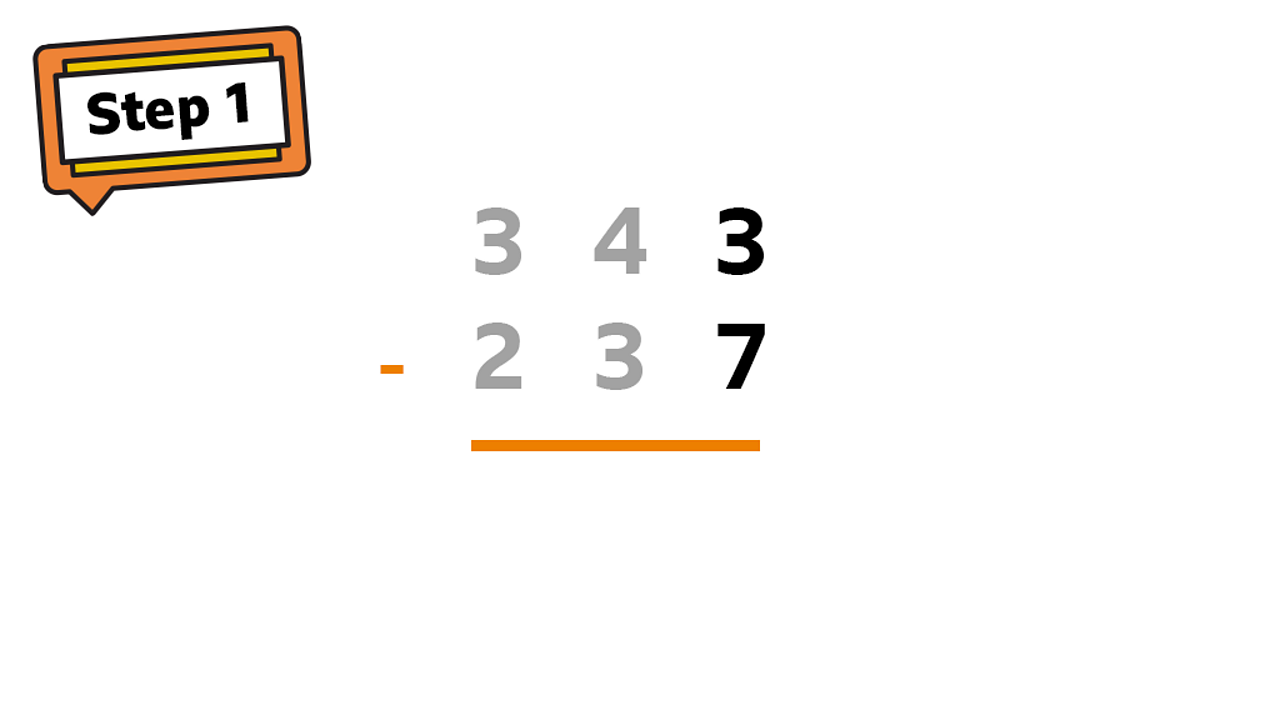Start from the ones column: 3 - 71 of 5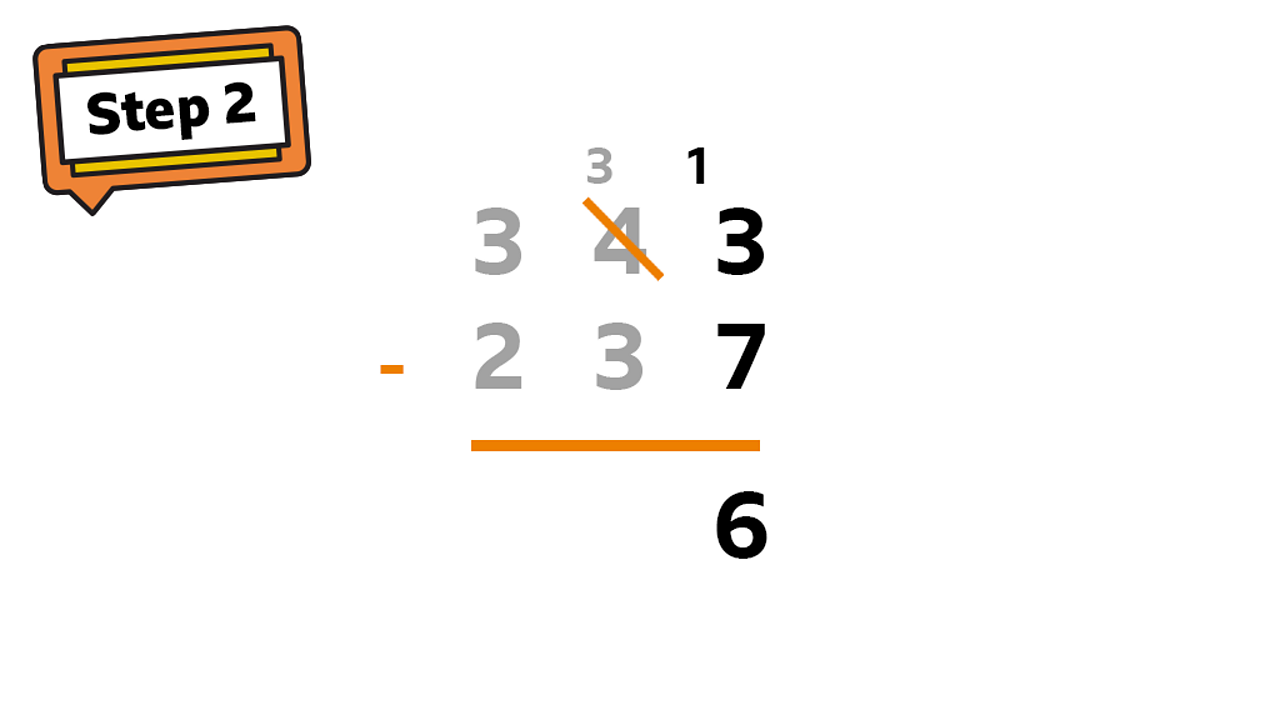It is not possible to subtract 7 from 3 so exchange one ten from the tens column for ten units: 13 - 7 = 62 of 5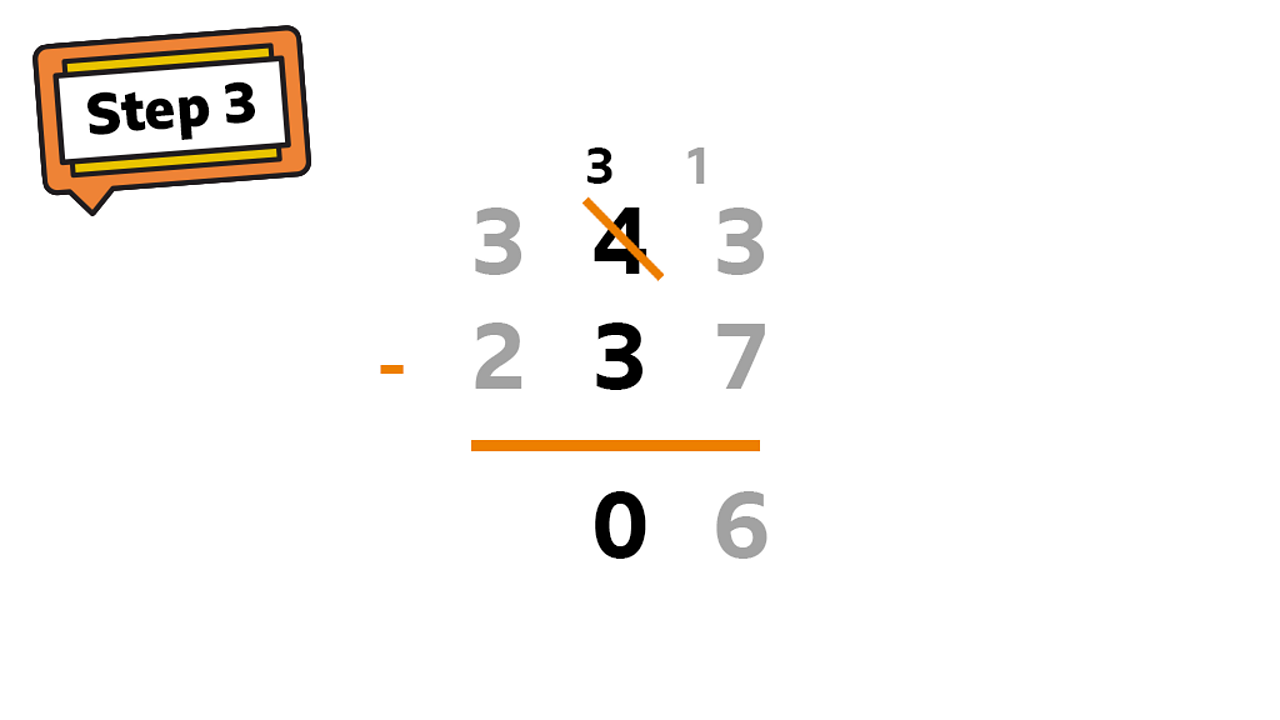In the tens column, one 10 has been exchanged so 40 becomes 30: 30 – 30 = 03 of 5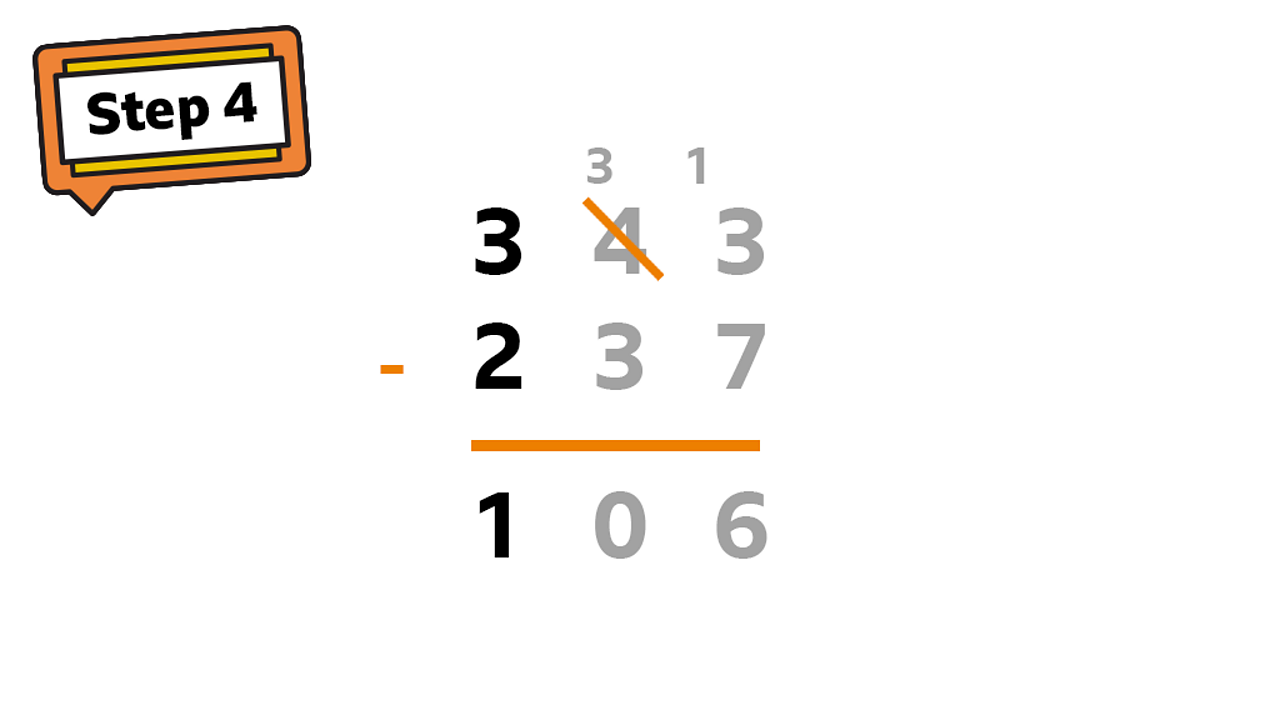In the hundreds column: 300 - 200 = 100. 1 is written in the hundreds column as this is worth 1004 of 5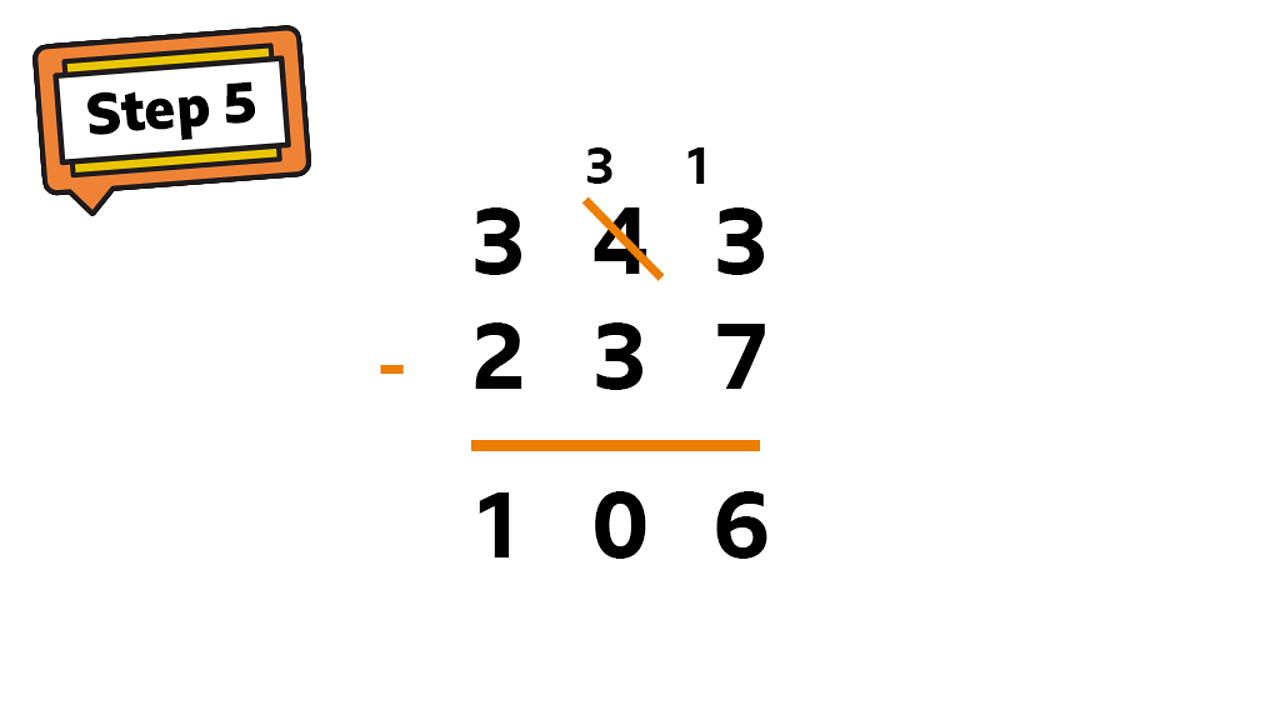The finished subtraction - 1065 of 5

## Example 3:

If you can subtract two three-digit numbers from each other, then you can use the same method to subtract two four-digit numbers!

You use the exact same method no matter how many digits are in the numbers.

Take a look at this example:

## Checklist

• Use the column method to help subtract large numbers.
• Separate the numbers into their place value of ones, tens, hundreds and thousands.
• Start on the right with the ones column first.